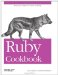# Sorting an Array by Frequency of Appearance

Problem

You want to sort an array so that its least-frequently-appearing items come first.

Solution

Build a histogram of the frequencies of the objects in the array, then use it as a lookup table in conjunction with the sort_ by method.

The following method puts the least frequently-appearing objects first. Objects that have the same frequency are sorted normally, with the comparison operator.

```	module Enumerable
def
sort_by_frequency
histogram = inject(Hash.new(0)) { |hash, x| hash[x] += 1; hash}
sort_by { |x| [histogram[x], x] }
end
end

[1,2,3,4,1,2,4,8,1,4,9,16].
sort_by_frequency
# => [3, 8, 9, 16, 2, 2, 1, 1, 1, 4, 4, 4]
```

Discussion

The sort_by_frequency method uses sort_by, a method introduced in Recipe 4.5 and described in detail in Recipe 4.6. The technique here is a little different from other uses of sort_by, because it sorts by two different criteria. We want to first compare the relative frequencies of two items. If the relative frequencies are equal, we want to compare the items themselves. That way, all the instances of a given item will show up together in the sorted list.

The block you pass to Enumerable#sort_by can return only a single sort key for each object, but that sort key can be an array. Ruby compares two arrays by comparing their corresponding elements, one at a time. As soon as an element of one array is different from an element of another, the comparison stops, returning the comparison of the two different elements. If one of the arrays runs out of elements, the longer one sorts first. Here are some quick examples:

```	[1,2] <=> [0,2] # => 1
[1,2] <=> [1,2] # => 0
[1,2] <=> [2,2] # => -1
[1,2] <=> [1,1] # => 1
[1,2] <=> [1,3] # => -1
[1,2] <=>  # => 1
[1,2] <=>  # => -1
[1,2] <=> [0,1,2] # => 1
[1,2] <=> [] # => 1
```

In our case, all the arrays contain two elements: the relative frequency of an object in the array, and the object itself. If two objects have different frequencies, the first elements of their arrays will differ, and the items will be sorted based on their frequencies. If two items have the same frequency, the first element of each array will be the same. The comparison method will move on to the second array element, which means the two objects will be sorted based on their values.

If you don't mind elements with the same frequency showing up in an unsorted order, you can speed up the sort a little by comparing only the histogram frequencies:

```	module Enumerable
def sort_by_frequency_faster
histogram = inject(Hash.new(0)) { |hash, x| hash[x] += 1; hash}
sort_by { |x| histogram[x] }
end
end

[1,2,3,4,1,2,4,8,1,4,9,16].sort_by_frequency_faster
# => [16, 8, 3, 9, 2, 2, 4, 1, 1, 4, 4, 1]
```

To sort the list so that the most-frequently-appearing items show up first, either invert the result of sort_by_frequency, or multiply the histogram values by1 when passing them into sort_by:

```	module Enumerable
def sort_by_frequency_descending
histogram = inject(Hash.new(0)) { |hash, x| hash[x] += 1; hash}
sort_by { |x| [histogram[x] * -1, x]}
end
end

[1,2,3,4,1,2,4,8,1,4,9,16].sort_by_frequency_descending
# => [1, 1, 1, 4, 4, 4, 2, 2, 3, 8, 9, 16]
```

If you want to sort a list by the frequency of its elements, but not have repeated elements actually show up in the sorted list, you can run the list through Array#uniq after sorting it. However, since the keys of the histogram are just the distinct elements of the array, it's more efficient to sort the keys of the histogram and return those:

```	module Enumerable
def sort_distinct_by_frequency
histogram = inject(Hash.new(0)) { |hash, x| hash[x] += 1; hash }
histogram.keys.sort_by { |x| [histogram[x], x] }
end
end

[1,2,3,4,1,2,4,8,1,4,9,16].sort_distinct_by_frequency
# => [3, 8, 9, 16, 2, 1, 4]
```

• Recipe 4.5, "Sorting an Array"
• Recipe 5.12, "Building a Histogram"Ruby Cookbook (Cookbooks (OReilly))
ISBN: 0596523696
EAN: 2147483647
Year: N/A
Pages: 399